It’s World Statistics Day! To honor the theme of the day, the JMP User Community is having conversations about the importance of trust in statistics and data. And we want to hear from you! Tell us the steps you take to ensure that your data is trustworthy.
Choose Language Hide Translation Bar
Data table tools, part 3: Numbering rows within groups to get flexible rankings

Have you ever had a data set where you had to perform tasks similar to either of these:

• From a tall table, containing multiple measurements by multiple tools — recorded at irregular intervals — create a subset table with only the kth-most recent measurement for each tool/operator combination.
• For a given metric, find the top (or bottom) k occurrences within each group; an example is listing which three products in each region generate the most revenue, or the lowest profit margin.

If so, you're not alone. We get requests pretty regularly for advice on how to tackle problems like this. Since each of these tasks requires ranking things, it is natural for JMP users to look to the rank ( ) function in JSL, and that is certainly an option. The issue is that the rank ( ) function requires the column by which the table’s rows are to be ranked to be explicitly specified, and when we explore data, we often want to experiment with a variety of rankings, without creating multiple new columns or editing column formulas.

By using the Col Cumulative Sum( ) function in JMP 13 creatively, we can rank the rows flexibly and dynamically: to produce a different ranking  we just sort by a different variable.

To illustrate, consider Car Physical Data.jmp, which contains nominal and continuous data about cars. It is installed in the JMP sample data folder, which you can access by selecting Help > Sample Data Library.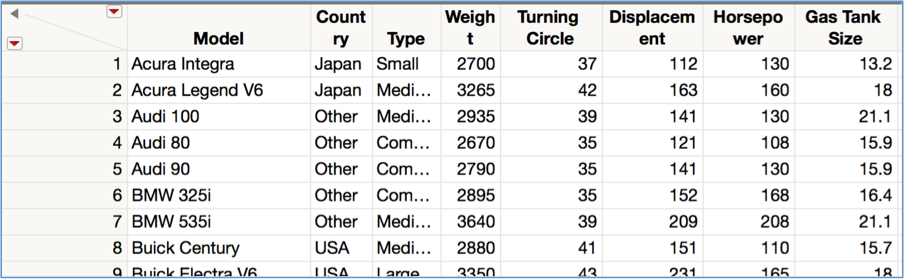Below, I’ve added a column (highlighted) to compute the cumulative number of cars, grouped by country, that occur in or before the given row. Notice that as we proceed down the rows of the table, the cars with Country = Japan are numbered sequentially, as are the cars for Country = USA and Country = Other.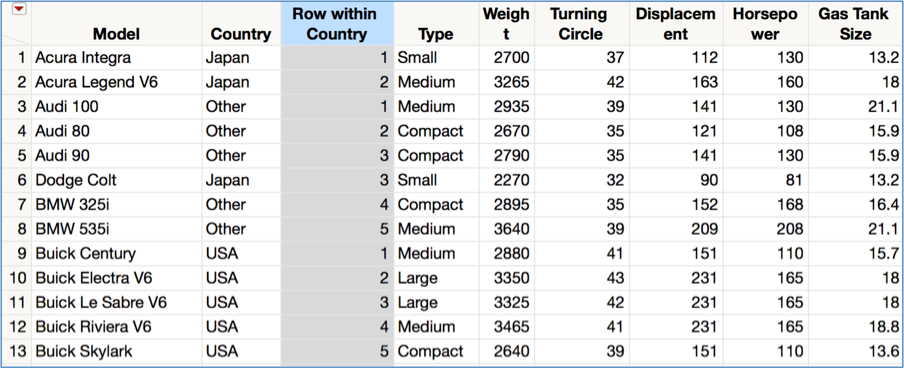This approach is powerful because to answer the question “which are the five lightest cars in each country?” we must only do the following:

• Sort the table by Weight, in ascending order, keeping the original table.
• Select the cells where the ranking column is <= 5.
• Subset these rows if desired.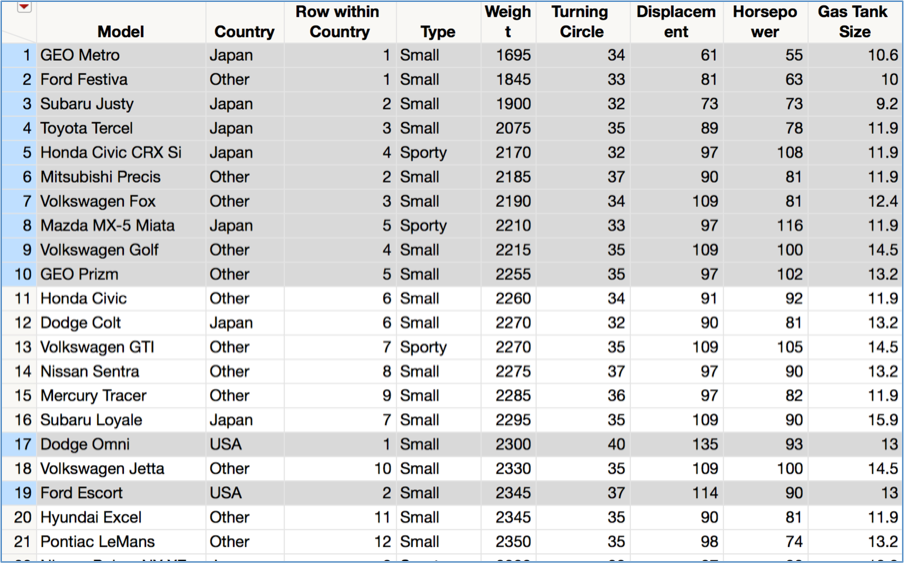This approach is also flexible because if we decide we now must find the three most powerful cars from each country, we simply sort by Horsepower in descending order and select cells where the ranking is less than or equal to three.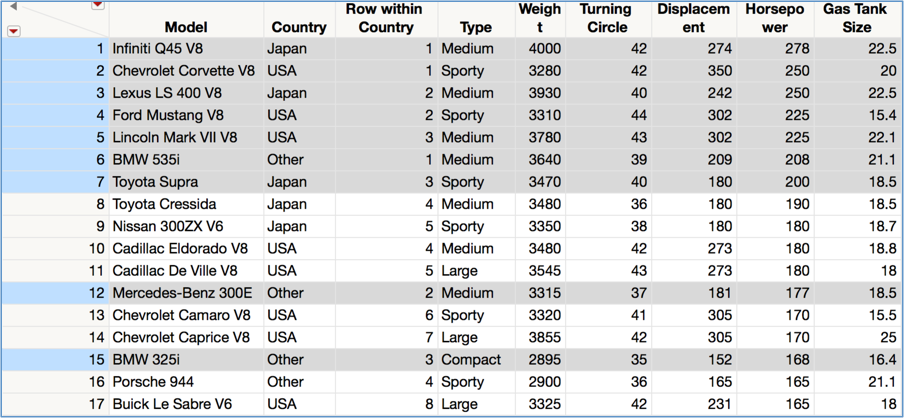The formula in the ranking column is simple (below), but you don’t have to write it down: Using the Data Table Tools add-in, even non-scripters can easily add a column like this to any table, using any combination of grouping columns.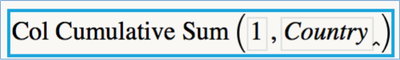Once you run the add-in, you just need to select the table and grouping columns you want, and click the "Create column" button. That's it!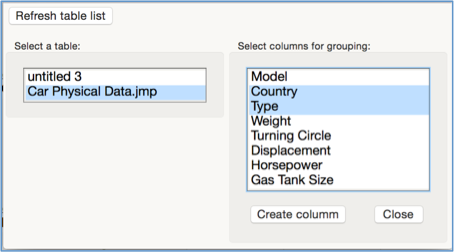The Data Table Tools add-in can be downloaded free from the JMP File Exchange — a free SAS profile is required.

Note: This blog is third in a series exploring the various features of the Data Table Tools add-in.

Article Labels

There are no labels assigned to this post.

Level I

Hello is it possible to do this with JMP 12?  Thank youStaff

Hello,

The Col Cumulative Sum function made its debut in JMP 13. Without it, you might try something like this, although current data table() will give issues when the table with this formula is not the current one. Note that if you want to use more than a single column to group, you will have to concatenate the multiple columns, and base the formula off that new column.

Cheers,

``````Names Default To Here( 1 );
dt = open("\$Sample_Data\Car Physical Data.jmp");
dt << New Column( "Rnk",
formula(
dtCur = Current Data Table();
ct = :country[Row()];
Contains( dtCur << get rows where( :country == ct ), Row() );
)
);

``````
Level I

That worked great thank you! I'm running this script immediately post SQL pull so current data table() works great.

And yes I do have more than one column to group (4 columns total) so creating a new column with the 4 columns concatendated worked perfectly.  The only thing is I'm working off data tables with 5000-6000 rows of data and there are about 780 grouped items I'm ranking so it takes JMP a minute or so to generate the Rnk column - but it works!

Thank you again!

Level IV

Prior to JMP13, this post covered how to do a rolling sum.  This post does not cover doing it by groups, but it's not too hard to extend the concept to that.

https://community.jmp.com/t5/Discussions/how-to-get-cumulative-sum-data/td-p/6766Staff

Specifically, jaredgj, try the formula:

k = row();

Summation( i = 1, k, If( :Country[i] == :Country[k], 1, 0 ) );

I'd forgotten about the summation function. It will be much faster than the other function since getting rows, as the other formula does, is time-consuming.

Cheers,